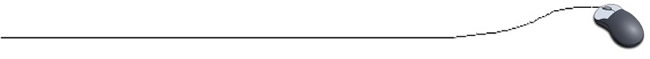Java Math Class and Methods

We have seen that Java contains a set of built-in math operators that perform simple mathematical operations such as addition, subtraction, multiplication, division and modulus. Java also contains the Java Math class which contains "methods" for dealing with more advanced mathematical computations.

Math is located in the java.lang package.
The full class name is java.lang.Math.
Remember, NO importing is needed to utilize the java.lang package.

Sample ways to engage these new methods:
 double amount = Math.round(12.344); //assigns the return value System.println(Math.sqrt(15)); // prints the returned square root of 15

These methods are used by invoking the name of the class (Math), followed by a dot (.), followed by the name of the method and any needed parameters.

 Method Declaration Purpose Basic Math Methods (Functions): Math.abs(x) int abs (int x) returns the absolute value of an integer. Also available for work with double or float. Math.ceil(x) double ceil (double x) rounds up to a whole number. ceil (11.2) rounds to 12.0 (not normal rounding) Math.floor(x) double floor (double x) rounds down to a whole number. floor(11.5) rounds to 11.0 (not normal rounding) Math.hypot(a,b) double hypot (double a, double b) calculates the hypotenuse (c) of a right triangle where a and b are the legs. Math.max(a,b) int max (int a, int b) returns the greater of the two integers passed. Also available for work with double or float. Math.min(a,b) int min (int a, int b) returns the lesser of the two integers passed. Also available for work with double or float. Math.PI double PI () returns a value close to the actual mathematical π Math.pow(x,y) (exponent) double pow (double x, double y) calculates x to the power of y. If x is negative, y must be an integer. If x is zero, y must be a positive integer. Math.random(x) (read more here) double random (double x) returns a random floating point number between 0 and 1. Math.round(x) double round (double x) rounds a double to the nearest integral using normal math rounding (either up or down). Math.sqrt(x) double sqrt (double x) calculates the positive square root of x. (x is >= 0) Exponential and Logarithmic Methods: Math.exp(x) double exp (double x) returns e raised to the power of x where e is Euler's e = 2.71828... Math.log(x) double log (double x) returns the natural logarithm of x, base e Math.log10(x) double log10 (double x) returns the logarithm of x, base 10 Trigonometric Methods: All work in radians rather than degrees. Math.cos(x) double cos (double x) returns the cosine of x in radians Math.sin(x) double sin (double x) returns the sine of x in radians Math.tan(x) double tan (double x) returns the tangent of x in radians Math.acos(x) double acos (double x) (x is between 1 and -1) returns the arc cosine of x (the angle whose cosine is x) Math.asin(x) double asin (double x) (x is between 1 and -1) returns the arc sine of x (the angle whose sine is x) Math.atan(x) double atan (double x) returns the arc tangent of x (the angle whose tangent is x) Math.atan2(y,x) double atan2 (double y, double x) converts rectangular coordinates (y, x) to polar coordinates (r, theta) Math.toDegrees (double angrad) double toDegrees (double a) converts an angle in radians to degrees Math.toRadians (double angdeg) double toRadians (double a) converts an angle in degrees to radians

Examples:

int ans1;
double ans2;
ans1 = Math.abs(-4); // ans1 equals 4
ans2 = Math.abs(-3.6); //ans2 equals 3.6

ans2 = Math.pow(2.0,3.0); //ans2 equals 8.0
ans2 = Math.pow(25.0,0.5); //ans2 equals 5.0

ans1 = Math.max(30,50); //ans1 equals 50
ans2 = Math.min(2.4, 5.3); //ans2 equals 2.4

ans2 = Math.round(5.66); //ans2 equals 6.0int